HSPT Math : Problem Solving

Example Questions

Example Question #591 : Problem Solving

Evaluate: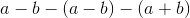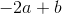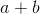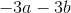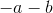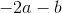Explanation:

In order to combine like terms correctly, it is necessary to simplify every term and eliminate the parentheses.   Double negatives equal a positive sign.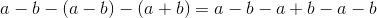Combine like terms.  The answer is:Example Question #592 : Problem Solving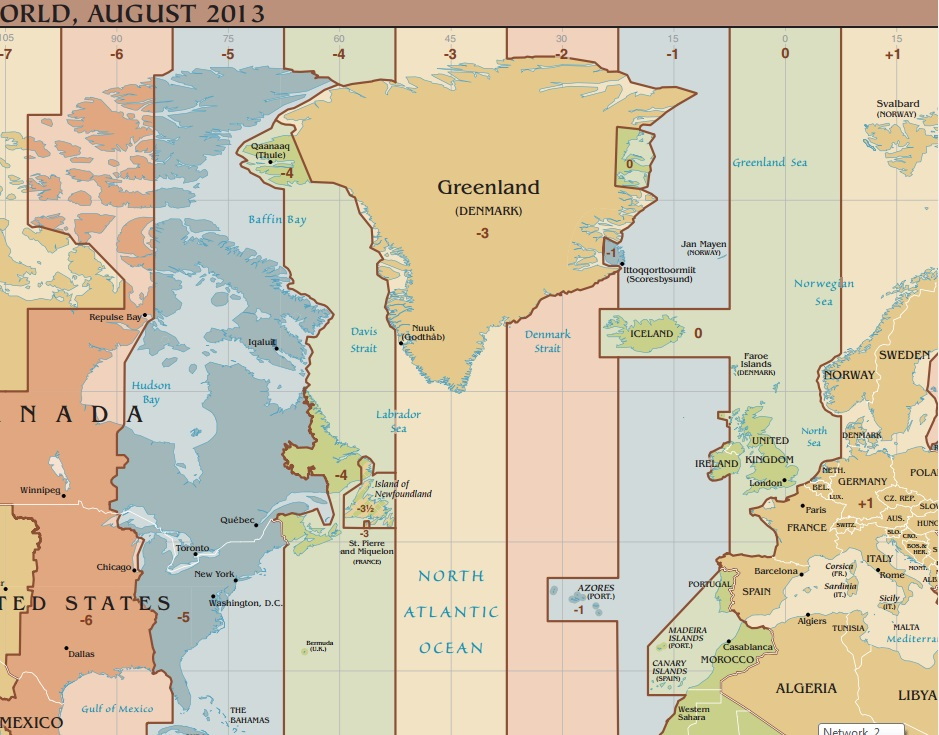Note: Public domain map from CIA World Factbook.

Refer to the above time zone map. The numbers along the top represent the difference, in hours, between the given time zone and Greenwich Mean Time (the time zone for the United Kingdom).

Which of the following expressions gives the time difference, in hours, between Paris and Dallas?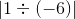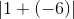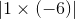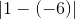Explanation:

A difference is the result of a subtraction. The time difference between the two cities will be the result of subtracting the numbers along the top of the map for their respective time zones, and taking the absolute value of the difference. The numbers for Paris and Dallas, respectively, are 1 and, so the difference in hours between their times is.

Example Question #593 : Problem SolvingNote: Public domain map from CIA World Factbook.

Refer to the above time zone map. The numbers along the top represent the difference, in hours, between the given time zone and Greenwich Mean Time (the time zone for the United Kingdom).

A flight took off from Winnipeg, Canada at 5:00 PM and took 7 hours to get to the Azores. What time was it in the Azores when it landed there?

7:00 PM

5:00 AM

5:00 PM

7:00 AM

5:00 AM

Explanation:

The time difference between Winnipeg and the Azores is the result of subtracting the numbers along the top of their respective timie zones, which areand: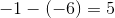The Azores is five hours ahead of Winnipeg, so when the flight takes off, since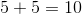,

it is 10:00 PM in the Azores.

Add the seven hours flight time, and take the result modulo 12: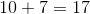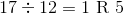The time is 5:00 AM in the Azores when the plane lands there.

Example Question #591 : Problem Solving

If you have a negative number and multiply it by another, the final answer is positive. What sign did the other number have?

None of the others

Does not matter

Negative

Cannot determine

Positive

Negative

Explanation:

The only way to get a positive product from multiplication is to multiply two positive numbers together or two negative numbers together.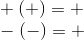If the first number was negative, the other number will also have to be negative.

Example Question #591 : Problem Solving

According to regulations, the maximum radius of a (spherical) bowling ball is 7.11 centimeters. What does that make the maximum surface area, to the nearest square centimeter?

(Note: disregard the holes)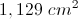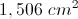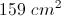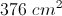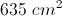Explanation:

Set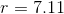and use the formula for the surface area of a sphere: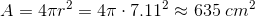Example Question #1 : Cylinders

An upright cylinder with a height of 30 and a radius of 5 is in a big tub being filled with oil. If only the top 10% of the cylinder is visible, what is the surface area of the submerged cylinder?

345π

300π

295π

325π

270π

295π

Explanation:

The height of the submerged part of the cylinder is 27cm. 2πrh + πr2 is equal to 270π + 25π = 295π

Example Question #1 : Cylinders

The diameter of the lid of a right cylindrical soup can is 5 in. If the can is 12 inches tall and the label costs $0.00125 per square inch to print, what is the cost to produce a label for a can? (Round to the nearest cent.) Possible Answers:$1.18

$0.24$0.29

$0.16$0.08

$0.24 Explanation: The general mechanics of this problem are simple. The lateral area of a right cylinder (excluding its top and bottom) is equal to the circumference of the top times the height of the cylinder. Therefore, the area of this can's surface is: 5π * 12 or 60π. If the cost per square inch is$0.00125, a single label will cost 0.00125 * 60π or $0.075π or approximately$0.24.

Example Question #1 : How To Find The Surface Area Of A Cylinder

Aluminum is sold to a soup manufacturer at a rate of $0.0015 per square inch. The cans are made so that the ends perfectly fit on the cylindrical body of the can. It costs$0.00125 to attach the ends to the can. The outer label (not covering the top / bottom) costs $0.0001 per in2 to print and stick to the can. The label must be 2 inches longer than circumference of the can. Ignoring any potential waste, what is the manufacturing cost (to the nearest cent) for a can with a radius of 5 inches and a height of 12 inches? Possible Answers:$0.45

$0.84$0.57

$0.91$0.77

$0.84 Explanation: We have the following categories to consider: <Aluminum Cost> = (<Area of the top and bottom of the can> + <Lateral area of the can>) * 0.0015 <Label Cost> = (<Area of Label>) * 0.0001 <Attachment cost> = 2 * 0.00125 =$0.0025

The area of ends of the can are each equal to π*52 or 25π. For two ends, that is 50π.

The lateral area of the can is equal to the circumference of the top times the height, or 2 * π * r * h = 2 * 5 * 12 * π = 120π.

Therefore, the total surface area of the aluminum can is 120π + 50π = 170π.  The cost is 170π * 0.0015 = 0.255π, or approximately $0.80. The area of the label is NOT the same as the lateral area of the can. (Recall that it must be 2 inches longer than the circumference of the can.) Therefore, the area of the label is (2 + 2 * π * 5) * 12 = (2 + 10π) * 12 = 24 + 120π. Multiply this by 0.0001 to get 0.0024 + 0.012π = (approximately)$0.04.

Therefore, the total cost is approximately 0.80 + 0.04 + 0.0025 = $0.8425, or$0.84.

Example Question #1 : How To Find The Surface Area Of A Cube

A room has dimensions of 18ft by 15ft by 9ft. The last dimension is the height of the room. It has one door that is 3ft by 7ft and two windows, each 2ft by 5ft. There is no trim to the floor, wall, doors, or windows. What is the total exposed wall space?

1093ft2

553 ft2

1134ft2

594 ft2

2389ft2

553 ft2

Explanation:

If broken down into parts, this is an easy problem. It is first necessary to isolate the dimensions of the walls. If the room is 9 ft high, we know 18 x 15 designates the area of the floor and ceiling. Based on this, we know that the room has the following dimensions for the walls: 18 x 9 and 15 x 9. Since there are two of each, we can calculate the total area of walls - ignoring doors and windows - by doubling the sum of these two areas:

2 * (18 * 9 + 15 * 9) = 2 * (162 + 135) = 2 * 297 = 594 ft2

Now, we merely need to calculate the area "taken out" of the walls:

For the door: 3 * 7 = 21 ft2

For the windows: 2 * (2 * 5) = 20 ft2

The total wall space is therefore: 594 – 21 – 20 = 553 ft2

Example Question #2131 : Hspt Mathematics

A room has dimensions of 23ft by 17ft by 10ft. The last dimension is the height of the room. It has one door that is 2.5ft by 8ft and one window, 3ft by 6ft. There is no trim to the floor, wall, doors, or windows. If one can of paint covers 57 ft2 of surface area. How many cans of paint must be bought to paint the walls of the room.

15

13

18

11

14

14

Explanation:

If broken down into parts, this is an easy problem. It is first necessary to isolate the dimensions of the walls. If the room is 10ft high, we know 23 x 17 designates the area of the floor and ceiling. Based on this, we know that the room has the following dimensions for the walls: 23 x 10 and 17 x 10. Since there are two of each, we can calculate the total area of walls - ignoring doors and windows - by doubling the sum of these two areas:

2 * (23 * 10 + 17 * 10) = 2 * (230 + 170) = 2 * 400 = 800 ft2

Now, we merely need to calculate the area "taken out" of the walls:

For the door: 2.5 * 8 = 20 ft2

For the windows: 3 * 6 = 18 ft2

The total wall space is therefore: 800 – 20 – 18 = 762 ft2

Now, if one can of paint covers 57 ft2, we calculate the number of cans necessary by dividing the total exposed area by 57: 762/57 = (approx.) 13.37.

Since we cannot buy partial cans, we must purchase 14 cans.